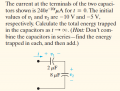# Frusturating question about energy in a capacitor

#### GreenMan357

Joined Apr 3, 2015
8
My question is whether is the sum of the energies on each capacitor is equal to the energy on the equivalent capacitor? (this is in respect to capacitors in series however it could extend to those in parallel as well). Here is the problem I am working on. I have worked it using the hint and got the right answer but can't for the life of me get the same energy using the equivalent capacitance. Since the equivalent capacitor should be identical to the two capacitors should it not also have the same energy?#### GreenMan357

Joined Apr 3, 2015
8

#### WBahn

Joined Mar 31, 2012
26,398
The problem is in the premise.

You need to understand what the formula for equivalent series capacitance means and what it doesn't mean. The formula describes capacitors that are initially uncharged and that, because of this, always end up with the same charge on them.

As soon as you start out with charged capacitors (that don't have the same charge), then the premise fails. To see that this is the case, let's take such a configuration and put it in a black box and then discharge it through a resistor. Once things settle down we will see no voltage at the terminals and we will conclude that the capacitor is discharged and thus has no energy stored in it. But in reality both capacitors will be charged in such a way that their voltages are equal and opposite and thus both capacitors will still contain energy.

•Hypatia's Protege
I get it now. Thank you very much.